MORE IN Structural Analysis 2
VTU Civil Engineering (Semester 5)
Structural Analysis 2
June 2015
Total marks: --
Total time: --
INSTRUCTIONS
(1) Assume appropriate data and state your reasons
(2) Marks are given to the right of every question
(3) Draw neat diagrams wherever necessary

1 (a) Draw the influence line diagram for bending moment at any given section of a simply supported beam.
4 M
1 (b) Show that for maximum bending moment at any section of simply supported girder traversed by a moving uniformly distributed load shorter than the girder span, the section should divide the uniformly distributed load in the same ratio as it divides the girder span.
6 M
1 (c) A uniformly distributed load of kN/m and 5m long sides across a beam of 15m long simply supported at its both ends. Determine Max Bending moment and shear at a section 6m from left hand support.
10 M

2 Analyze the continuous beam shown in Fig Q2 by slope deflection method. Draw B.M.D.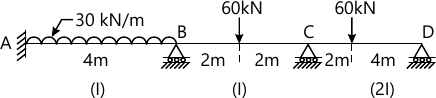20 M

3 Analyze the frame shown in Fig. Q3 by moment distribution methods. Draw B.M.D.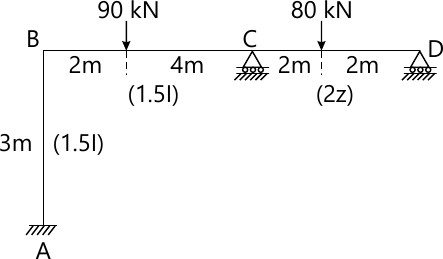20 M

4 Analyze the frame shown below by moment distribution methods. Draw B.M.D. (refer Fig, Q No 4).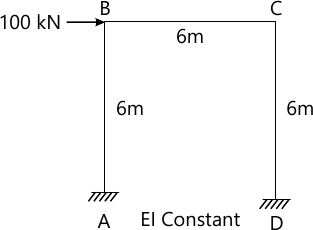20 M

5 Analyze the frame shown in Fig Q5 by taking advantages of symmetry. Draw B.M.D.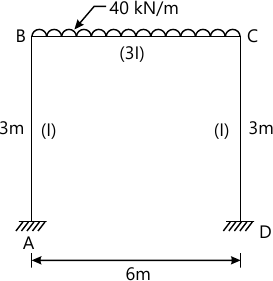20 M

6 Analyze the frame shown in Fig Q6 by using Flexibility matrix method. Use system approach.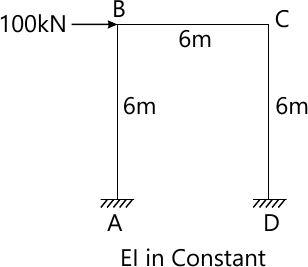20 M

7 Find the displacement components along the system coordinates for the frame shown in Fig. Q7 using stiffness method (use system approach).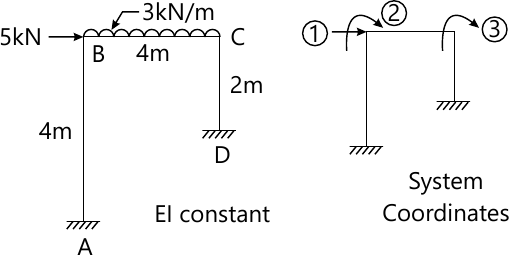20 M

8 (a) Define the following terms:
i) Free Vibration
ii) Forced Vibration
iii) Periodic motion
iv) Natural frequency
v) Damping.
10 M
8 (b) Determine the natural frequency and Time period of the system as shown in Fig Q8 (b). Take E=2.1×105 N/mm2 and I=13×106 mm4.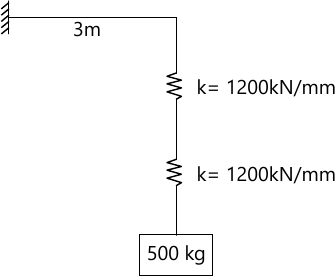10 M

More question papers from Structural Analysis 2